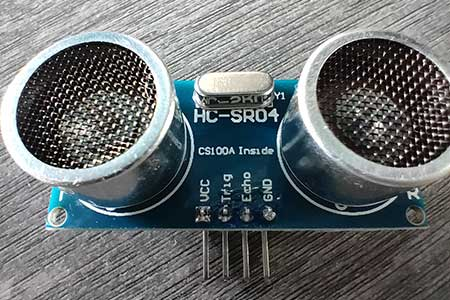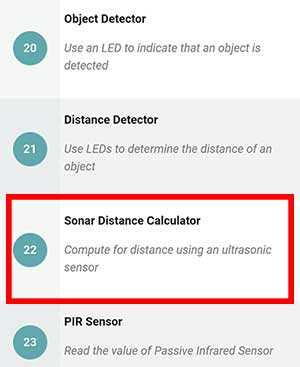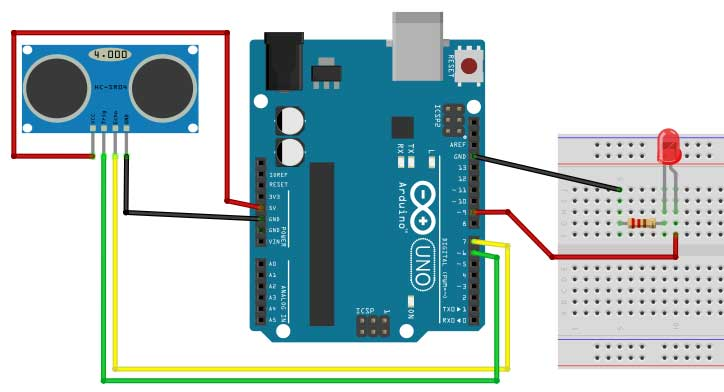# Using the HC-SR04 Ultrasonic Sensor to Detect Objects

With sound or ultrasonic sensors, a high-frequency signal beyond the range of human hearing is generated by an oscillator. The signal is then passed to a transducer which emits the signal in varying ultrasonic frequencies. If there are objects within the range of the sensor, the reflected sound will then be processed by the sensor. The delay between the transmission and reception of the sound gives an indication as to how far or how near an object is.

The ultrasonic sensor is another type of proximity sensor. These proximity sensors all use the basic detection principle. The sensor emits a signal which will bounce back once it detects an obstacle. The sensor will read the bounced signal and compare it to the original signal emitted. If there is a difference between those signals, the sensor will then consider that it has encountered an obstacle.In this project, we will be using the HC-SR04 ultrasonic sensor module. This sensor can detect objects from 2 cm to 400 cm, or roughly, 1 inch to 13 feet. The sensing angle is 15 degrees or less. Its operating voltage is 5 volts.

The pins of the HC-SR04 are VCC, TRIG, ECHO, and GND. The VCC pin connects to the 5V pin of the Arduino, GND connects to the GND pin. You can attach the TRIG and ECHO pin to any digital pin, except 0 and 1.

# PROJECT 1: Sonar Distance CalculatorTo test our HC-SR04 ultrasonic sensor, we will make Project 22: Sonar Distance Calculator of the Arduino Intro app. This project will simply print out the distance of an object in the Serial Monitor. The distance will be expressed in inches.In our circuit, the TRIG pin is connected to pin 6 and the ECHO pin is connected to pin 7. VCC goes to 5V and GND goes to GND.

Now let’s go to the code.

``````

//compute for distance using ultrasonic sensor

const  int trigger=6;
const  int echo=7;
float  distance;
float dist_inches;

void setup(){
Serial.begin(9600);
pinMode(trigger,OUTPUT);
pinMode(echo,INPUT);
}

void loop(){
// Trigger the sensor to start measurement
// Set up trigger
digitalWrite(trigger,LOW);
delayMicroseconds(5);

//Start Measurement
digitalWrite(trigger,HIGH);
delayMicroseconds(10);
digitalWrite(trigger,LOW);

// Acquire and convert to inches
distance=pulseIn(echo,HIGH);
distance=distance*0.0001657;
dist_inches=distance*39.37;

// print the value on the serial monitor
Serial.print(dist_inches);
Serial.println(" inches");
delay(200);
}
``````

First, we need to declare our variables.

``````	const  int trigger=6;
const  int echo=7;``````

The first variable is const int trigger=6; The const keyword stands for constant which means that we can no longer change the value of the trigger variable. However, this is not really necessary, you can still write it as int trigger=6; .The same can be said about the echo variable. The trigger variable is needed so we can control when the sensor will emit the sound signal. The echo variable will enable us to receive the reflected sound signal.

``````	float  distance;
float dist_inches;``````

Next are the distance and dist_inches variables. They are assigned as float data types and not int. All variables with a float data type can accept whole numbers with decimal parts. We will use float since the signal that we will be getting from the HC-SR04 ultrasonic sensor is encoded in decimal form. Later on in the code, we will convert the distance value to inches and it will be saved in the dist_inches variable.

``````		Serial.begin(9600);
pinMode(trigger,OUTPUT);
pinMode(echo,INPUT);``````

Remember that we will be using the Serial Monitor to print the distance of an object in inches. So, in the setup function, we write Serial.begin(9600). Next, we need to set the trigger variable as an OUTPUT pin and the echo variable as an INPUT pin. Again, trigger enables the sensor to send the signal, while, echo enables it to receive the reflected signal.

Next, we go to the loop function.

``````		digitalWrite(trigger,LOW);
delayMicroseconds(5);``````

First, we need to clear the trigger pin so we can start sending out the signal. We do this by writing digitalWrite(trigger,LOW); followed by delayMicroseconds(5);

``````		digitalWrite(trigger,HIGH);
delayMicroseconds(10);
digitalWrite(trigger,LOW);``````

Next, we will start sending out the ultrasonic signal. This can be achieved by writing digitalWrite(trigger,HIGH); followed by delayMicroseconds(10); and lastly, digitalWrite (trigger, LOW);

``		distance=pulseIn(echo,HIGH);``

Now, we will expect the signal to bounce back once an object is detected. So we need to prepare the echo pin to receive that signal. To do this, we need to write distance=pulseIn(echo, HIGH);. This means that whenever a reflected signal is received, it will be saved to the distance variable. The HC-SR04 sensor will express the signal in decimal form.

As of this time, the value of our distance variable is still meaningless as it’s just a series of numbers. Therefore, we need to convert it into something that we can measure easily.

``````		distance=distance*0.0001657;
dist_inches=distance*39.37;``````

The next part of the code will convert the value of the distance variable into inches. The formula is simple, we just multiply the distance variable by 0.0001657, which is a constant. Finally, we multiply the result by the constant 39.37. The result will then be saved to the dist_inches variable.

``````		// print the value on the serial monitor
Serial.print(dist_inches);
Serial.println(" inches");
delay(200);``````

Finally, we are ready to print the actual distance in inches in the Serial Monitor. We write Serial.print(dist_inches); followed by Serial.println(“ inches”). This technique enables us to print the actual number of inches followed by a space and the word inches. They will be written on the same line.

A delay of 200 milliseconds is added which means that the next reading will happen after 200 milliseconds have passed.

# PROJECT 2: Ultrasonic Detector

Now, let us try to turn on an LED once an object is detected by our ultrasonic sensor.For the code, we simply add an if-else statement after we get the distance value. Our code will now look like this:

``````  //turn on an LED based on distance measured by
//ultrasonic sensor

int pinled=9;
const  int trigger=6;
const  int echo=7;
float  distance;
float dist_inches;

void setup(){
Serial.begin(9600);
pinMode(trigger,OUTPUT);
pinMode(echo,INPUT);
pinMode(pinled,OUTPUT);
}

void loop(){
// Trigger the sensor to start measurement
// Set up trigger
digitalWrite(trigger,LOW);
delayMicroseconds(5);

//Start Measurement
digitalWrite(trigger,HIGH);
delayMicroseconds(10);
digitalWrite(trigger,LOW);

// Acquire and convert to inches
distance=pulseIn(echo,HIGH);
distance=distance*0.0001657;
dist_inches=distance*39.37;

if(dist_inches < 6){ //if distance of object is less than 6 inches
digitalWrite(pinled,HIGH);
}
else{
digitalWrite(pinled,LOW);
}

}``````

To adjust the distance of the object, simply change the number on this line:

``if(dist_inches < 6){ //if distance of object is less than 6 inches``

Ultrasonic sensors are a great addition to your Arduino projects. However, there may be some things that can interfere with the signal. The problem with sound sensors is that the resolution of sound decreases with distance. It’s also possible to get false readings since the physical properties of objects may change the frequency of the sound that is reflected or may cause the sound to be absorbed completely. Nonetheless, ultrasonic sensors remain a popular choice among robotics enthusiasts.